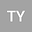loading page

Parameter Estimation in Uncertain Heat Equations
•## Abstract

Uncertain heat equations are aimed to model the variation of temperature in a given region over time under uncertain influence. Parameter estimation is an important and significant topic in uncertain heat equations because after we construct a uncertain heat equation according to the specific problem to model a dynamic system, it is natural that the uncertain heat equation contains unknown parameters such as the unknown thermal diffusivity and unknown parameters of strength of heat source. For that matter, this paper first employs the moment method to estimate unknown parameters in uncertain heat equations. To show the process of parameter estimation, two numerical examples are given.